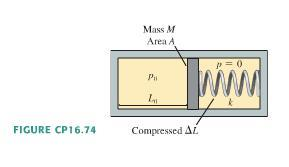# Piston & Gas Law Problem

• bcjochim07

## Homework Statement

The closed cylinder in the figure has a tight-fitting but frictionless piston of mass M. The piston is in equilibrium when the left chamber has pressure p0 and length L0 while the spring on the right is compressed by delta L. The spring constant of the spring is k.

a)What is delta L in terms of p0, L0, A, M, and k?

b) Find an expression for the net force on the piston. Assume all motions of are slow enough for the gas to remain at the same temp.

## The Attempt at a Solution

a) I didn't include all of the variables, so it seems that this part is too simple. Here's what I did: F=pA

so the force of the gas on the piston = the force of the spring on the piston

p0A=kdeltaL
deltaL= p0A/k

b) p1v1=p2v2

p0(L0)(A) = p1(L0+x)(A)

p1= p0L0/(L0 + x)

So the force is pA = Ap0L0/(L0+x) is this right?